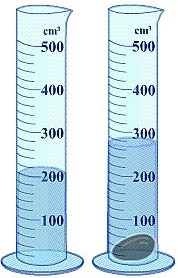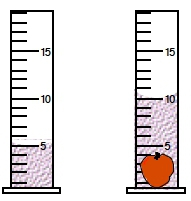Start typing, then use the up and down arrows to select an option from the list.# GOB Chemistry

Learn the toughest concepts covered in your GOB - General, Organic, and Biological Chemistry class with step-by-step video tutorials and practice problems.

Matter and Measurements

# Density of Non-Geometric Objects

The Density of non-geometric objects can be determined using water displacement.

## Calculating Density

1

#### concept

Density of Non-Geometric Objects Concept and Example

The video is coming soon
we're not gonna take a look at how to calculate the density of non geometric objects. Now we're gonna say here that water displacement is the amount of water moved out of the way. When an object is totally submerged, we're gonna say water displacement can be used to determine the volume of non geometric objects. At this point, we know how to calculate density overall, and we've looked at the density of spheres, cubes and cylinders. When we talk about non geometric objects, we're talking about objects with irregular shapes. Now, if we take a look here at this first example, it's as calculate the volume of the water displaced by the submerging of the object. Here we're told that the volume of the given cylinders are in milliliters. So if we take a look at the first beaker on the left were going to say where the water touches the line, that's our meniscus. And where it touches at the base of this curve, that's its volume. And if we take a look, we're gonna say up here is one middle leader. So each of these hash marks is 1/10 place, so this is 0.1 point 2.3 point 4.5 point 6.7 So we have 0.7 middle leaders as the volume off the liquid liquid by itself. Now, when I take this non geometric object and throw it in there, the volume increases because now it's not only the volume of the initial liquid, but it's also the volume of the object as well. So we're gonna say their volume together. So this is one 0.4 mL, and the meniscus touches this line here, so that would be 1.1. So we'd say 1.1 middle leaders is the volume off the liquid plus object. Okay, so we have to do at this point, it's attract them from one another, and the difference what's left behind would have to represent the volume of the object itself. So we subtract these two, so that means we have 0.4 mL, and that would be the volume of my non geometric object. So I just realized here water displacement. We're just taking the object, and we're totally submerging it in. The water has to be completely covered by the water or the liquid, and the new volume is the volume of both the object plus the initial liquid. Usually a lot of these. We're talking about water. So let's just assume that this liquid is water. Okay, so following this will help us determine the volume of the inanimate object. Now that we've seen this example, let's move on and take a look at the practice question.
2
Problem

A piece of unknown solid weighs approximately 0.045 lbs. When a scientist places it in a glass beaker the water level increases from 200 mL to 260 mL. What is the density of the unknown solid in g/mL?3
Problem

If an irregularly shaped apple possesses a density of 0.96 g/cm3, what is its mass in milligrams? (The volume of the given cylinders are in mL).